SOLAR SYSTEM DATA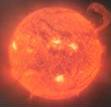THE SUN
The Sun is the largest and most massive body in the solar system. Located at the centre, all other objects in the solar system are, by definition, gravitationally bound to the Sun. The Sun is composed mostly of hydrogen and helium (92% H and 8% He by numbers of atoms) although almost any element you can name is present. Helium was first discovered on the Sun, by virtue of the spectral lines that appear in the Sun's light. Helium was later found on the Earth in natural gas deposits (mostly in the USA and Russia). Technetium, an element with atomic number of 43, does not occur naturally on the Earth (although it is now made in nuclear reactors), but is also present in the Sun. Although we refer to a solar surface (termed the photosphere), the Sun has no solid surface, and this so-called surface is simply the layer from which visible light and heat is radiated into space.

The Sun does not spin or rotate as a solid body. The equator rotates faster than do the polar regions. Different layers inside the Sun also rotate at different rates than the layers immediately above them. This differential rotation is of course only possible because the Sun is a gaseous body (a big ball of gas). Even with the very high estimated pressures at the solar core, the central temperature is high enough to keep the matter in a gaseous state.

Models and measurements from helioseismology lead us to believe that the Sun is divided internally into 3 different regions. The core (about 20% of the solar diameter) is where the nuclear fusion reactions occur that power the Sun. The radiative zone extends from the core out to a radius of about 70% of the Sun. This region is so dense that we believe it can take up to one million years for the particles of heat and light (photons) generated in the core to work their way through it. The outer 30% of the solar diameter is the convection zone where motions are very turbulent. The top of this zone is the photosphere.

The Sun has a constantly changing magnetic field, which is to believed to be generated at the base of the convection zone by the motions there. This magnetic field, twisted by the differential rotation of the Sun, is believed to produce sunspots on the solar surface together with solar activity such as flares and coronal mass ejections that can be observed with special filters.

The solar atmosphere is divided into a lower atmosphere (the chromosphere) and the outer atmosphere (the corona). The corona extends out through the solar system as what we term the solar wind. The coronal temperature near the Sun is about 2 million degrees (K).

APPROXIMATE SOLAR PARAMETERS

Diameter = 1,400,000 km ( = 109 times the Earth's diameter )
Mass = 2 x 1030 kg ( 330,000 Earth masses )
Volume = 1.4 x 1027 m3 ( 1.3 million times Earth's volume)
Average density = 1400 kg m-3 ( 1.4 times the density of water)
Surface gravity = 270 m s-2 ( 27 x Earth's gravity )
Surface temperature = 6,000 K
Surface pressure = 1 kPa ( 1/100 Earth's sea level pressure of 101 kPa)
Core temperature = 15 million K ( estimated from models )
Core pressure = 2.3 x 1013 kPa
Core density = 150,000 kg m-3
Average rotation rate = once every 27 days ( varies with latitude )
Luminosity = 3.8 x 1026 W
Fuel consumption = 5 millions tons of hydrogen per second
Fuel cycle - nuclear fusion ( 4 H atoms fuse to form a He atom )
Sunspot cycle period = 11 years
Age = 5,000 million years ( estimated from models )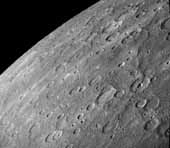MERCURY
Mercury is the closest planet to the Sun and is classified as a terrestrial or Earth-like planet. It is difficult to observe because it is always less than 28o from the Sun. Mariner 10 was the only spacecraft to visit Mercury (1974-5) until the recent pass of Messenger in 2008. The Mariner passes, supplemented by Earth-based radar have been the main sources of information about the planet.

The surface of Mercury is covered by craters, and one large impact feature, named Caloris, is spread over 1300 km and has 'weird' terrain at its antipodal point. Of the four terrestrial planets (Mercury, Venus, Earth and Mars), only Mercury and the Earth have a global magnetic field.

ORBITAL PARAMETERS FOR MERCURY

Mean distance from the Sun = 57.9 million km (0.387 AU)
Orbital eccentricity = 0.206
Orbital inclination to the ecliptic = 7.01 degrees
Orbital period = 87.969 days
Mean orbital velocity = 47.9 km/sec
Advance in perihelion = 10 minutes of arc per century

PLANETARY PARAMETERS

Mass = 3.30 x 1023 kg
Mean density = 5430 kg/m3
Gravity = 3.7 m/sec2 = 0.38 g (g = Earth gravity)
Escape velocity = 4.25 km/sec
Synodic rotation period (mean) = 115.9 days
Sidereal rotation period = 58.6 days (= 2/3 of orbital period)
Surface temperature range = 100 - 700 K
Inclination of equator to orbit = 1o (approx)
Mean solar constant = 9940 W/m2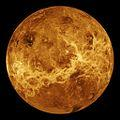VENUS
Venus is the second planet to the Sun, Earth's closest planetary neighbour, and often the brightest object in the night sky on a moonless night. It appears in the evening or morning sky, and has a maximum angular distance from the Sun of 47 degrees (as observed from Earth). Venus has a massively dense atmosphere which excludes visual/optical observation of any surface features, even to an orbital satellite. These clouds give the planet a very high albedo, which contributes to its brightness. Radar on orbiting spacecraft has been used to produce a detailed map of Venusian topography. Venus has a very similar radius and density to that of the Earth, but the surface pressure and temperature make it a very hostile place, not only to living organisms but even to spacecraft, which have only survived for time scales measured in minutes.

ORBITAL PARAMETERS FOR VENUS

Mean distance from the Sun = 108.2 million km (0.723 AU)
Orbital eccentricity = 0.007
Orbital inclination to the ecliptic = 3.39 degrees
Orbital period = 224.70 days
Mean orbital velocity = 35.0 km/sec

PLANETARY PARAMETERS

Mass = 4.87 x 1024 kg
Mean density = 5243 kg/m3
Gravity = 8.9 m/sec2 = 0.91 g (g = Earth gravity)
Escape velocity = 10.4 km/sec
Synodic rotation period (mean) = 116.8 days
Sidereal rotation period = 243.0 days (retrograde)
Surface temperature range = 650 - 750 K (estimates)
Inclination of equator to orbit = 177o
Mean solar constant = 2614 W/m2
Surface atmospheric pressure = 9,200 kPa
Surface atmospheric density = 65 kg/m3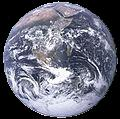EARTH
Earth is the third planet from the Sun and home to the only life so far known in the Universe. It has a global magnetic field, which gives rise to a magnetosphere which shields the planet and its atmosphere from the normal solar wind. The atmosphere is around 20% oxygen and 80% nitrogen with less than 1% other gases. Most of the atmosphere lies below 10 km altitude, but minute traces exist to several hundred kilometres, allowing the formation of an ozone layer (~30km) which provides protection against most UV radiation and an ionosphere (80 to 500 km) which not only absorbs solar X-radiation but also allows long distance radio communication. Seventy percent of the planet is covered with water. The solid planet is divided into a crust, a mantle and an outer and inner core. The Earth is the first planet from the Sun to have a natural satellite, the Moon.

ORBITAL PARAMETERS FOR EARTH

Mean distance from the Sun = 149.6 million km (1.000 AU)
Orbital eccentricity = 0.017
Orbital inclination to the ecliptic = 0.00 degrees
Orbital period = 365.26 days
Mean orbital velocity = 29.8 km/sec

PLANETARY PARAMETERS

Mass = 5.97 x 1024 kg
Mean density = 5515 kg/m3
Gravity = 9.8 m/sec2 = 1.00 g (g = Earth gravity)
Escape velocity = 11.2 km/sec
Synodic rotation period (mean) = 24.00 hours
Sidereal rotation period = 23.935 hours
Surface temperature range = 180 - 330 K
Inclination of equator to orbit = 23.45o (approx)
Mean solar constant = 1376 W/m2
Surface atmospheric pressure = 101 kPa
Surface atmospheric density = 1.23 kg/m3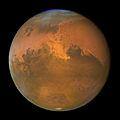MARS
Mars is the fourth planet from the Sun and the last of the terrestrial class (rocky) planets. Mars has no global magnetic field and it is thought that this absence probably allowed the solar wind to erode any previous substantial atmosphere down to the low density and pressure observed today. The Martian atmosphere is predominantly carbon dioxide and thin high cloud has been observed. The humidity is close to zero. Dust devils and global dust storms have been observed frequently. The high eccentricity of the orbit leads to large changes in solar insolation which vie with axial tilt for climate control in the lower latitude regions of Mars. The mean density of the planet is substantially lower than that of the other three terrestrial planets, which all lie between 5 and 5.5 gm/cc. Mars has two small moons which are probably captured asteroids.

ORBITAL PARAMETERS FOR MARS

Mean distance from the Sun = 227.9 million km (1.52 AU)
Orbital eccentricity = 0.093
Orbital inclination to the ecliptic = 1.85 degrees
Orbital period = 686.98 days
Mean orbital velocity = 24.1 km/sec

PLANETARY PARAMETERS

Mass = 6.42 x 1023 kg